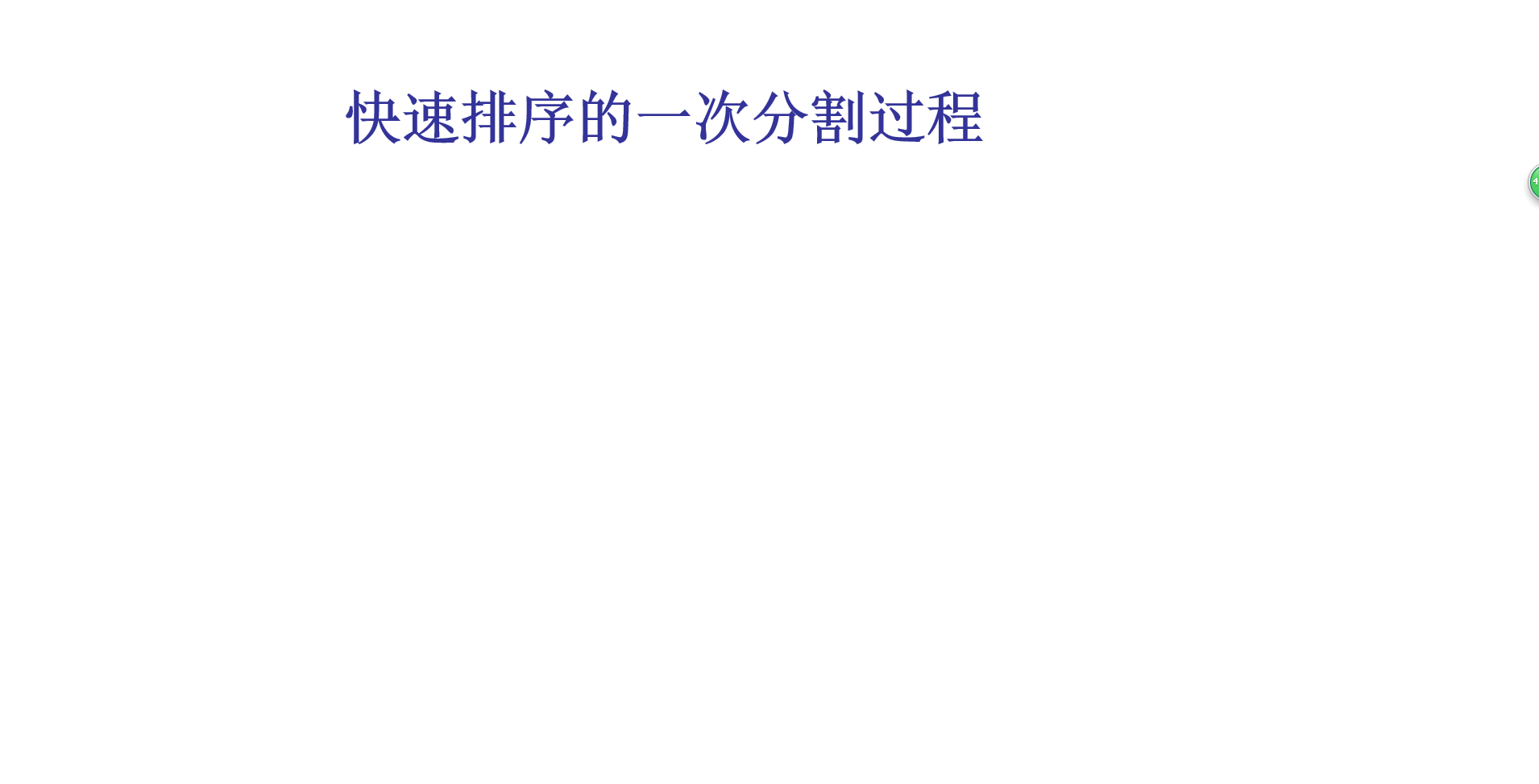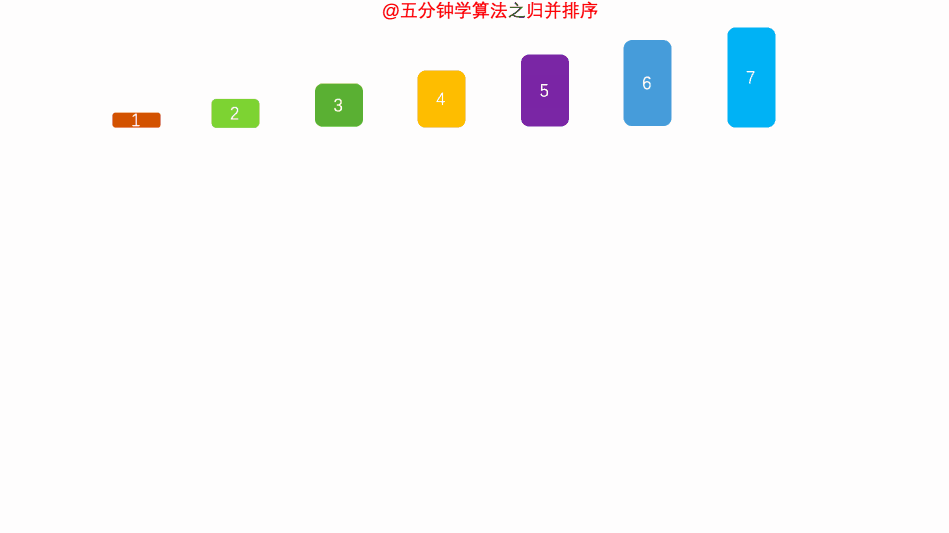# 从零开始学数据结构和算法 (五) 分治法 (二分查找、快速排序、归并排序)Android架构

}

return -(low+1);//low+1 表示找不到时停在了第 low+1 个元素的位置

}

### 概念

• 快速排序（Quicksort）是对冒泡排序的一种改进。

### 动画演示### 代码实例

//快速排序 31 21 59 68 12 40

// x=31

public static

《Android学习笔记总结+最新移动架构视频+大厂安卓面试真题+项目实战源码讲义》浏览器打开：qq.cn.hn/FTe 免费领取

void quickSort(int[] array,int begin,int end){

if(end-begin<=0) return;

int x=array[begin];

int low=begin;//0

int high=end;//5

//由于会从两头取数据，需要一个方向

boolean direction=true;

L1:

while(low<high){

if(direction){//从右往左找

for(int i=high;i>low;i--){

if(array[i]<=x){

array[low++]=array[i];

high=i;

direction=!direction;

continue L1;

}

}

high=low;//如果上面的 if 从未进入，让两个指针重合

}else{

for(int i=low;i<high;i++){

if(array[i]>=x){

array[high--]=array[i];

low=i;

direction=!direction;

continue L1;

}

}

low=high;

}

}

//把最后找到的值 放入中间位置

array[low]=x;

//开始完成左右两边的操作

quickSort(array,begin,low-1);

quickSort(array,low+1,end);

}

### 概念

• 归并排序（MERGE-SORT）是建立在归并操作上的一种有效的排序算法,该算法是采用分治法（Divide and Conquer）的一个非常典型的应用。将已有序的子序列合并，得到完全有序的序列；即先使每个子序列有序，再使子序列段间有序。若将两个有序表合并成一个有序表，称为二路归并

### 算法思路

• 归并操作(merge)，也叫归并算法，指的是将两个顺序序列合并成一个顺序序列的方法。

### 动画演示### 上代码

@Test

public void test(){

int[] array=new int[]{2,1,6,4,3,9,8,10,7,5};

// merge(array,0,4,7);

mergeSort(array,0,array.length-1);

for (int i : array) {

System.out.print(i+" ");

}

}

public static void mergeSort(int array[],int left,int right){

if(left==right){

return;

}else{

int mid=(left+right)/2;

mergeSort(array,left,mid);

mergeSort(array,mid+1,right);

merge(array,left,mid+1,right);

}

}

// 0 4 7

// 1 2 5 9 === 3 4 10 11

public static void merge(int[] array,int left,int mid,int right){

int leftSize=mid-left;

int rightSize=right-mid+1;

//生成数组

int[] leftArray=new int[leftSize];

int[] rightArray=new int[rightSize];## 评论